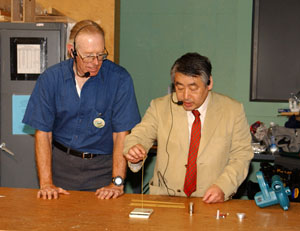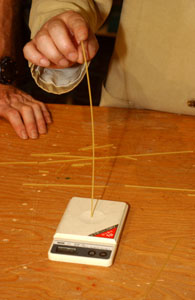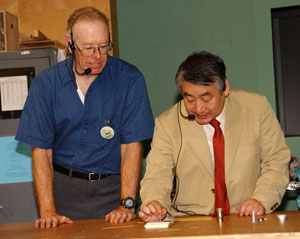Try This! Break Spaghetti

An exploration demonstrated by Dr. Yoji TakikawaDr. Takikawa pushes a piece of spaghetti down onto a scale until it breaks.

Introduction

Measure the force that it takes to break a piece of spaghetti and discover a mathematical pattern.

Material

A digital scale that will measure up to one kilogram ( 2 pounds)

4 pieces of spaghetti

Try This

Turn on the scale, place one piece of spaghetti vertically in the center of the pan of the scale.A long piece of spaghetti on the scale.

Step 1. A whole piece of spaghetti.

Press down on the top of the piece of spaghetti with the palm of your hand slowly increase the pressure as you measure the force applied until the spaghetti breaks. Notice hat as you push on the spaghetti it bends to one side. Notice the force at which the spaghetti breaks. For example the full length piece of spaghetti may break when the scale reads 50 grams. (Scientific note about grams and force)

Note, if the bottom of the piece of spaghetti slides off to one side tape a piece of sticky tape, sticky-side-up to the center of the scale pan. Put one end of the spaghetti in the center of the tape and it will not slide to one side.

Step 2. A half piece of spaghetti.

Try breaking a half a piece of spaghetti. Notice that it takes a higher force to break the shorter piece. in fact the scale reads 200 grams when the spaghetti breaks. It takes 4 times the force to break a half piece of spaghetti.

Step 3. A quarter piece of spaghettiIt takes much more force to bend a short piece of spaghetti.

Try breaking a piece of spaghetti one quarter the length of the first piece. This short piece may break when the scale reads 800 grams.

Repeat these steps several times and average the results for each length to get more meaningful scientific data.

What's Going On?

Consider the spaghetti as a beam.

Notice that when you cut the length of a beam in half it takes four times the force to break it. (When the beam is not clamped at each end.)

This is an inverse square law. The force it takes to break a beam, F, is proportional to the inverse square of the length of the beam, L.

In mathematical terms F a 1/L^2

This law of buckling beams was discovered by Leonard Euler. In his honor this failure mode is called Euler buckling.

So What?

This law shows why it is difficult to build tall buildings. Gravity pulls down on the building taller buildings are easier to break. Dr. Takikawa noted that 3000 years ago people built pyramids 756 feet high. Today the highest building is the CNN Tower in Canada. It is only 1500 feet high. In 3000 years we have only made buildings two times higher.

Going Further

Try different lengths of spaghetti, try 1/3 a whole piece.

Try two pieces side by side at the same time.

Try two pieces held them together in the middle with a piece of tape. Try three pieces.

About kilograms and force. Scales are calibrated to measure mass in kilograms or grams. They actually measure the force of gravity on a mass. The force on one kilogram is found by multiplying the mass by the acceleration of gravity which is 9.8 m/s^2. So the force on one kilogram is really 9.8 newtons. Since this activity is about ratios of forces we can use units of mass and get the correct answers.

Average the results. Break four pieces of spaghetti one at a time. Notice the readings on the scale at which each piece breaks. Add the four values together and divide by four.

For example if the four readings are 200,220,180, 190 grams

Then the average is (200+220+180+190)/4 = 198 grams (Where I have rounded the result)

Paul Doherty